# Local maximum from the left implies left-hand derivative is nonnegative if it exists

This article describes a test that can be used to determine whether a point in the domain of a function gives a point of local, endpoint, or absolute (global) maximum or minimum of the function, and/or to narrow down the possibilities for points where such maxima or minima occur.
View a complete list of such tests

## Statement

Suppose$f$ is a function and$\! c$ is a point of local maximum from the left for$c$, i.e., there exists a value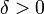$\delta > 0$ such that$f(x) \le f(c)$ for all$x \in (c - \delta,c)$ (i.e.,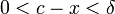$0 < c - x < \delta$).

Further, suppose that the left-hand derivative of$f$ at$c$ exists. Then, this left hand derivative is nonnegative, i.e., it is either positive or zero.

## Proof

Given:$f$ is a function and$\! c$ is a point of local maximum from the left for$c$, i.e., there exists a value$\delta > 0$ such that$f(x) \le f(c)$ for all$x \in (c - \delta,c)$, i.e.,$0 < c - x < \delta$.

To prove: If$f'_-(c)$, i.e., the left hand derivative of$f$ at$c$ exists, then it is positive or zero, i.e.,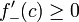$f'_-(c) \ge 0$.

Proof: The left hand derivative is the left hand limit of the difference quotient, i.e., it is given as:$\! f'_-(c) := \lim_{x \to c^-} \frac{f(x) - f(c)}{x - c}$

We proceed as follows:

Step no. Assertion Given data used Previous steps used Explanation
1 To take the limit as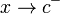$x \to c^-$, it suffices to restrict attention to$x \in (c - \delta,c)$, i.e., we only care about the behavior of$f$ on the immediate left of$c$ Follows from the definition of limit
2 For$x \in (c - \delta,c)$, the denominator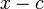$x - c$ is negative, and the numerator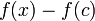$f(x) - f(c)$ is non-positive, i.e., it is either negative or zero$f(x) \le f(c)$ for$x \in (c -\delta,c)$. The denominator is negative because$x < c$. The numerator is positive or zero because$f(x) \le f(c)$ for$x \in (c -\delta,c)$.
3 For$x \in (c - \delta,c)$, the difference quotient$\frac{f(x) - f(c)}{x - c}$ is non-negative, i.e., it is either positive or zero. Step (2) Quotient of a number that is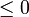$\le 0$ by a number that is$< 0$ must be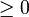$\ge 0$.
4 If$f'_-(c)$ exists, it must be non-negative Step (3) By Step (3),$f'_-(c)$ is the limit of an expression that's$\ge 0$. Hence, it must be$\ge 0$.

### Note on strictness

Note that even if we assume that$f$ is a point of strict local maximum from the left (i.e., that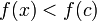$f(x) < f(c)$ for$x \in (c - \delta, c)$, we can only conclude that the left hand derivative at$c$, if it exists, is positive or zero. We cannot eliminate the possibility of its being zero.

The reason is as follows: we do know that for a strict local maximum from the left, the difference quotient with any point on the immediate left is positive. However, the limit of this quantity can still be zero, because the limit of a function taking positive values can be zero.

The fact that the zero case survives even in the strict situation is crucial to the observation that point of local extremum implies critical point.